# 2nd PUC Maths Previous Year Question Paper March 2018

Students can Download 2nd PUC Maths Previous Year Question Paper March 2018, Karnataka 2nd PUC Maths Model Question Papers with Answers helps you to revise the complete Karnataka State Board Syllabus and score more marks in your examinations.

## Karnataka 2nd PUC Maths Previous Year Question Paper March 2018

Time: 3 Hrs 15 Min
Max. Marks: 100

Instructions

• The question paper has five parts namely A, B, C, D, and E. Answer all the parts.
• Use the graph sheet for the question on Linear programming in Part – E

Part – A

Answer ALL the following questions: (10 × 1 = 10)

Question 1.
Define the bijective function.
Solution:
A function f: A → B is said to be bijective if it is both one-one and onto.

Question 2.
Write the principal value brach of cos-1 x.
Solution:
(o, π)Question 3.
Construct a 2 × 2 matrix, A = [aij], whose elements are given by, aij = $$\frac{i}{j}$$
Solution: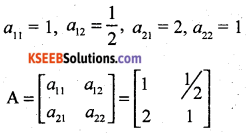Question 4.
If A is an invertible matrix of order 2 then find |A-1|.
Solution:
|A-1| = $$\frac{1}{|A|}$$

Question 5.
If y = $$e^{x^{3}}$$, find $$\frac{d y}{d x}$$
Solution:
$$\frac{d y}{d x}=e^{x^{2}} \cdot 3 x^{2}$$

Question 6.
Find: $$\int \frac{x^{3}-1}{x^{2}} d x$$
Solution: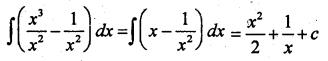Question 7.
Find the unit vector in the direction of the vector $$\vec{a}=\hat{i}+\hat{j}+2 \hat{k}$$
Solution: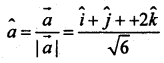Question 8.
If a line makes angle 90°, 60° and 30°with the positive direction of x, y and z-axis respectively, find its direction cosines.
Solution:
cos 90° = 0, cos 60° = $$\frac {1}{2}$$, cos 30° = $$\frac { \sqrt { 3 } }{ 2 }$$
dc’s are 0, $$\frac {1}{2}$$, $$\frac { \sqrt { 3 } }{ 2 }$$Question 9.
Define the optimal solution in a linear programming problem.
Solution:
Any feasible solution of LPP which maximizes or minimizes the objective function is called an optimal solution.

Question 10.
If P(A) = $$\frac{7}{13}$$, P(B) = $$\frac{9}{13}$$ and P(A ∩ B) = $$\frac{4}{13}$$, find P(A/B)
Solution: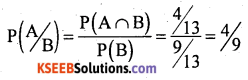Part – B

Answer any TEN questions: (10 × 2 = 20)

Question 11.
Let * be a binary operation on Q, defined by a * b = $$\frac{a b}{2}$$, ∀ a, b ∈ Q

Question 12.
sin($$\sin ^{-1} \frac{1}{5}+\cos ^{-1} x$$) = 1 then find the value of x.
Solution: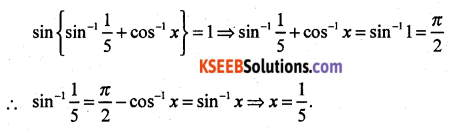Question 13.
Write the simplest from of $$\tan ^{-1}\left(\frac{\cos x-\sin x}{\cos x+\sin x}\right)$$, 0 < x < $$\frac{\pi}{2}$$
Solution:Question 14.
Find the area of the triangle whose vertices are (-2, -3), (3, 2) and (-1, -8) by using the determinant method.
Solution: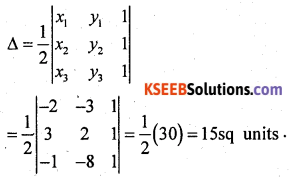Question 15.
Differentiate xsin x, x > 0 with respect to x.
Solution:
Let y = xsin x
Taking logarithm on both sides, we have
log y = sin x log x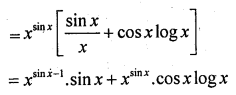Question 16.
Find $$\frac{d y}{d x}$$, if x2 + xy + y2 = 100
Solution:
x2 + xy + y2 = 100
Differentiating with respect to x.
2x + x $$\frac{d y}{d x}$$ + y(1) + 2y $$\frac{d y}{d x}$$ = 0
⇒ (x + 2y) $$\frac{d y}{d x}$$ = -(2x + y)
⇒ $$\frac{d y}{d x}=-\frac{(2 x+3)}{x+2 y}$$

Question 17.
Find the slope of the tangent to the curve y = x3 – x at x = 2.
Solution:
$$\frac{d y}{d x}$$ = 3x2 – 1
At x = 2, $$\frac{d y}{d x}$$ = 3(4) – 1 = 11 = slope of the tangent.

Question 18.
Integrate $$\frac{e^{{tan}^{-1}} x}{1+x^{2}}$$ with respect to x.
Solution:
$$\frac{e^{{tan}^{-1}} x}{1+x^{2}}$$Question 19.
Evaluate $$\int_{2}^{3} \frac{x d x}{x^{2}+1}$$
Solution: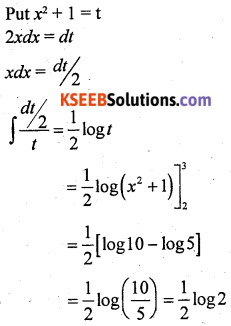Question 20.
Find the order and degree of the differential equation:Solution:
Order = 3, degree = 2.

Question 21.
Find the projection of Jhe vector $$\hat{i}+3 \hat{j}-7 \hat{k}$$ on the vector $$7 \hat{i}+\hat{j}+8 \hat{k}$$
Solution:Question 22.
Find the area of the parallelogram whose adjacent sides are determined by the vectors $$\vec{a}=3 \hat{i}+\hat{y}+4 \hat{k}$$ and $$\vec{b}=\hat{i}-\hat{j}+\hat{k}$$Question 23.
Find the angle between the planes whose vector equations are $$\vec{r} \cdot(2 \hat{i}+2 \hat{j}-3 \hat{k})=5$$ and $$\vec{r} \cdot(3 \hat{i}+3 \hat{j}-5 \hat{k})=3$$
Solution: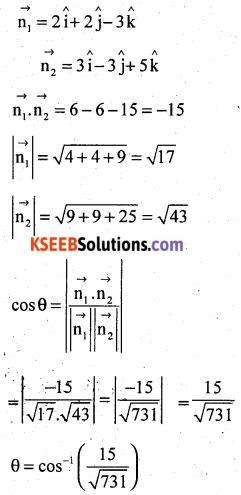Question 24.
A random variable X has the following probability distribution:
Determine:
(i) k
(ii) P(X ≥ 2)
Solution:
The probability distribution of X is(i) We know that $$\sum_{i=1}^{n} p i=1$$
Therefore 0.1 + k + 2k + 2k + k = 1
⇒ k = 0.15

(ii) P(you study at least two hours) = P(X ≥ 2)
= P(X = 2) + P(X = 3) + P(X = 4)
= 2k + 2k + k
= 5k
= 5 × 0.15
= 0.75
P(you study exactuly two hours) = P(X = 2)
= 2k
= 2 × 0.15
= 0.3
P(you study at most two hours) = P(X ≤ 2)
= P(X = 0) + P(X = 1) + P(X = 2)
= 0.1 + k + 2k
= 0.1 + 3k
= 0.1 +3 × 0.15
= 0.55

Part – C

Answer any TEN questions: (10 × 3 = 30)

Question 25.
Show that the relation R in the set A = {1, 2, 3, 4, 5} given by R = {(a, b) : |a – b| is even}, is an equivalence relation.
Solution:
|a – a| = |o| = 0 is even
(a, a) ∈ R.
∴ R is reflexive.
Let (a, b) ∈ R ⇒ |a – b| is even
⇒ |-(a – b)| is even
⇒ |(b – a)| is even
⇒ (b, a) ∈ R
∴ R is symmetric.
Let (a, b) ∈ R and (b, c) ∈ R
⇒ |a – b| is even and |b – c| is even
⇒ a – b is even
⇒ b – c is even
⇒ a – b + b – c is even
⇒ a – c is even
⇒ |a – c| is even
∴ (a, c) ∈ R.
∴ R is transitive
∴ R is an equivalence relation.Question 26.
Prove that $$2 \tan ^{-1} \frac{1}{2}+\tan ^{-1} \frac{1}{7}=\tan ^{-1} \frac{31}{17}$$ in the simplest form.
Solution: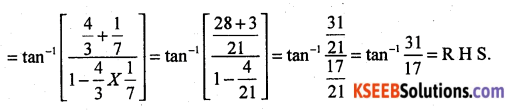Question 27.
By using elementary transformations, find the inverse of the matrix A = $$\left[\begin{array}{ll}1 & 3 \\2 & 7\end{array}\right]$$
Solution: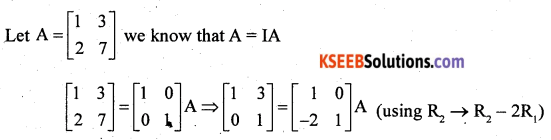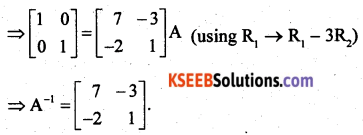Question 28.
If x = sin t, y = cos 2t then prove that $$\frac{d y}{d x}$$ = -4 sin t

Question 29.
Verify Rolle’s theorem for the function f(x) = x2 + 2, x ∈ [-2, 2]
Solution:
The function y = x2 + 2 is Continuous in {-2, 2} and differentiable in (-2, 2). Also f(-2) = f(2) = 6 and hence the value of f(x) at -2 and 2 coincide.
Rolle’s theorem states that there is a point c ∈ (-2, 2) where f'(c) = 0. Since f'(x) = 2x, we get c = 0. Thus at c = 0, we have f'(c) = 0 and c = 0 ∈ (-2, 2).

Question 30.
Find two numbers whose sum is 24 and whose product is as large as possible.
Solution:
Let one number be x. Then, the other number is (24 – x).
(∵ Sum of the two numbers is 24)
Let y denotes the product of the two numbers. Thus, we have
y = x(24 – x) = 24x – x2
On differentiating twice w.r.t. x, we get
$$\frac{d y}{d x}$$ = 24 – 2x and $$\frac{d^{2} y}{d x^{2}}$$ = -2
Now, put $$\frac{d y}{d x}$$ = 0
⇒ 24 – 2x = 0
⇒ x = 12
At x = 12, $$\frac{d^{2} y}{d x^{2}}$$ = -2 < 0
∴ By second derivative test, x = 12 is the point of local mxima of y. Thus, the product of the number is maximum when the numbers are 12 and 24 – 12 = 12.
Hence, the numbers are 12 and 12.Question 31.
Find: $$\int \frac{x d x}{(x+1)(x+2)}$$

Question 32.
Find: ∫ex sin x dx
Solution:
Take ex as the first function and sin x as second function. Then integrating by parts, we have
I = ∫ex sin x dx = ex (-cos x) + ∫ex cos x dx = -ex cos x + I1 (say) …… (1)
Taking ex and cos x as the first and second functions, respectively, in I1, we get
I = ex sin x – ∫ex sin x dx
Substituting the value of I1 in (i), we get
I = -ex cos x + ex sin x – 1 or 2I = ex (sin x – cos x)
Hence I = ∫ex sin x dx = $$\frac{e^{x}}{2}$$ (sin x – cos x) + C

Question 33.
Find the area of the region bounded by the curve y = x2 and the line y = 4.
Solution: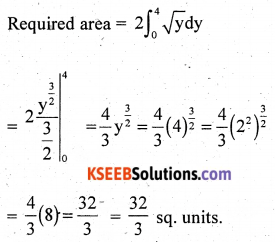Question 34.
Form the differential equation representing the family of curves y = a sin(x + b), where a, b are arbitrary constants.

Question 35.
Show that the position vector of the point P, which divides the fine joining the points A and B having position vectors $$\vec{a}$$ and $$\vec{b}$$ internally in the ratio m : n is $$\frac{m \vec{b}+n \vec{a}}{m+n}$$

Question 36.
Find x such that the fojir points A(3, 2, 1) B(4, x, 5), C(4, 2, -2) and D(6, 5, -1) are coplanar.
Solution:Question 37.
Find the equation of the plane through the intersection of the planes 3x – y + 2z – 4 = 0 and x + y + z – 2 = 0 and the point (2, 2, 1).Question 38.
A bag contains 4 red and 4 black balls, another bag contains 2 red and 6 black balls. One of the two bags is selected at random and a ball is drawn from the bag which is found to be red. Find the probability that the ball is drawn from the first bag.

Part – D

Answer any SIX questions: (6 × 5 = 30)

Question 39.
Lef R+ be the set of all non-negative real numbers. Show that the function f : R+ → [4, ∞) given by f(x) = x2 + 4 is invertible and write the inverse of f.
Solution: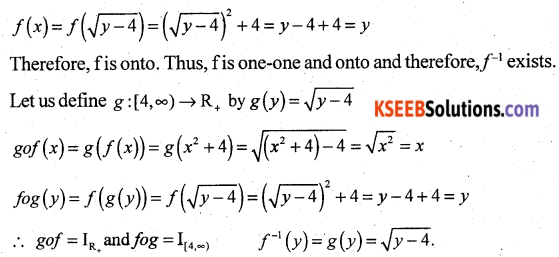Question 40.
If A = $$\left[\begin{array}{rrr}0 & 6 & 7 \\-6 & 0 & 8 \\7 & -8 & 0\end{array}\right]$$, B = $$\left[\begin{array}{lll}0 & 1 & 1 \\1 & 0 & 2 \\1 & 2 & 0\end{array}\right]$$, C = $$\left[\begin{array}{c}2 \\-2 \\3\end{array}\right]$$, calculate AC, BC and (A + B)C. Also verify that (A + B)C = AC + BC.
Solution: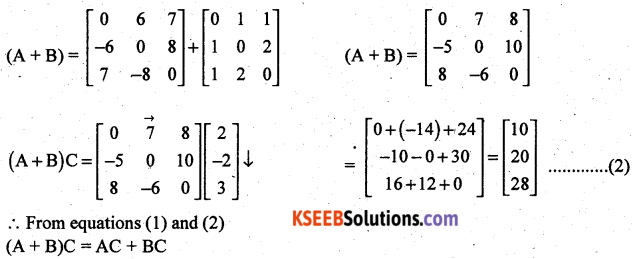Question 41.
Solve the following system of linear equations by matrix method.
x – y + 2z = 7, 3x + 4y – 5z = -5 and 2x – y + 3z = 12.

Question 42.
If y = (tan-1 x)2. Show that (x2 + 1)2 y2 + 2x(x2 + 1) y1 = 2.
Solution:Question 43.
Sand is pouring from a pipe at the rate of 12 cm3/s. The falling sand forms a cone on the ground is such a way that the height of the cone is always one-sixth of the radius of the base. How fast is the height of the sand cone increasing when the height is 4 cm?
Solution:
Let r be the radius, h be the height and V be the volume of the sand cone at any time t.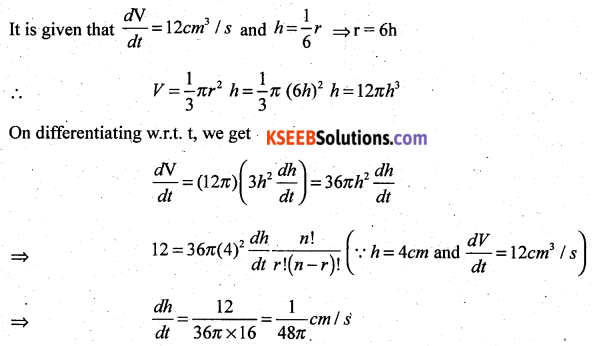Hence, when the height of the sand cone is 4 cm, its height is increasing at the rate of $$\frac{1}{48 \pi}$$ cm/s.Question 44.
Find the integral of $$\frac{1}{x^{2}+a^{2}}$$ with respect to x and hence find $$\int \frac{1}{x^{2}-6 x+13} d x$$
Solution: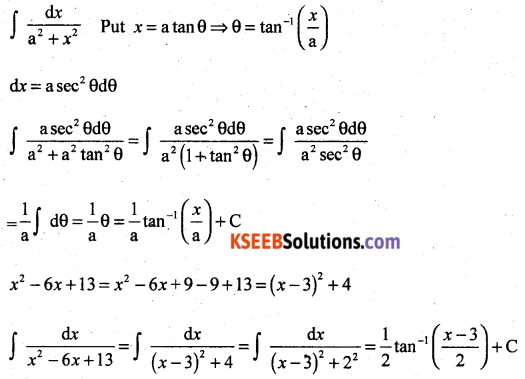Question 45.
Using integration find the area of the region bounded by the triangle whose vertices are (1, 0), (2, 2) and (3, 1).
Solution:
Area = Area of ∆ABD + Area of trapezium BDEC – Area of ∆AEC
Now equation of the sides AB, BD and CA are given by
y = 2(x – 1), y = 4 – x, y = $$\frac{1}{2}$$(x – 1) respectively
Hence, area of ∆Question 46.
Find the general solution of the differential equation x $$\frac{d y}{d x}$$ + 2y = x2 log x.
Solution: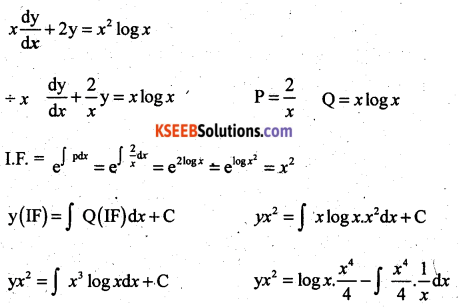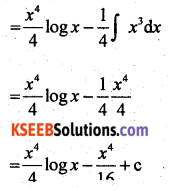Question 47.
Derive the equation of the line in space passing through two given points, both in vector and Cartesian form.
Solution:
Vector Form
Let $$\overrightarrow { a }$$ and $$\overrightarrow { b }$$ be the position vectors of two points A (x1, y1, z1) and B(x2, y2, z2) respectively that are lying on a line.
Let $$\overrightarrow { r }$$ be the position vector of an arbitrary point P(x, y, z), then P is a point on the line if and only if $$\overrightarrow{\mathrm{AP}}=\overrightarrow{\mathrm{r}}-\overrightarrow{\mathrm{a}}$$ and $$\overrightarrow{\mathrm{AB}}=\overrightarrow{\mathrm{b}}-\overrightarrow{\mathrm{a}}$$ are collinear vectors. Therefore, P is on the line if and only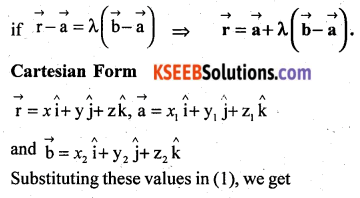Question 48.
If a fair coin is tossed 10 times, find the probability of
Solution:
Let X denote the number of heads in an experiment of 10 trials.Part – E

Answer any ONE question: (1 × 10 = 10)

Question 49(a).
Prove that $$\int_{0}^{a} f(x) d x=\int_{0}^{1} f(a-x) d x$$ and hence evaluate $$\int_{0}^{a} \frac{\sqrt{x}}{\sqrt{x}+\sqrt{a-x}} d x$$
Solution: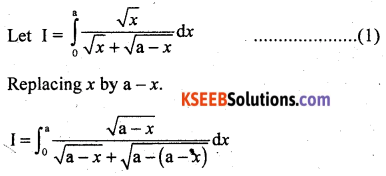Question 49(b).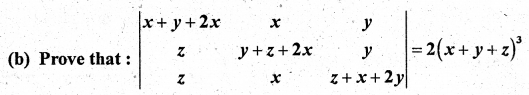Solution:Question 50(a).
Solve the following problem graphically:
Minimise and Maximise: z = 3x + 9y
Subject to the constraints:
x + 3y ≤ 60, x + y ≥ 10, x ≤ y, x ≥ 0, y ≥ 0.
Solution:
Let us graph the feasible region of the system of linear inequalities (2) to (5).
The coordinates of the corner point A, B, C and D are (0, 10), (5, 5), (15, 15) and (0, 20) respectively.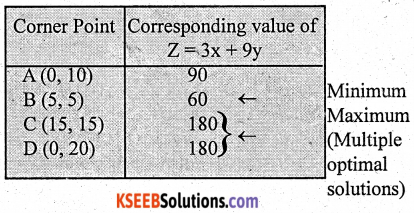We now find the minimum and maximum value of Z. From the table, we find that the minimum value of Z is 60 at point B (5, 5) of the feasible region.
The maximum value of Z on the feasible region occurs at the two comer points C(15, 15) and D(0, 20) and it is 180 in each case.Question 50(b).
Find the relationship between a and b so that function f defined by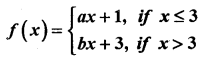is continuous at x = 3.
Solution:3a + 1 = 3b + 3 = 3a + 1
⇒ 3a + 1 = 3b + 3
⇒ 3a = 3b + 3 – 1
⇒ 3a = 3b + 2
⇒ a = b + $$\frac{2}{3}$$

error: Content is protected !!